M4 Macros for Electric Circuit Diagrams in L
A
T
E
X Documents
Dwight Aplevich
Contents, Version 9.6.4
1 Introduction . . . . . . . . . . . . . . . . . . . . . 1
2 Using the macros . . . . . . . . . . . . . . . . 2
2.1 Quick start . . . . . . . . . . . . . 2
2.1.1 Using m4 . . . . . . . . . . 3
2.1.2
Processing with dpic and
PSTricks or Tikz PGF . . . 3
2.1.3 Processing with gpic . . . . 3
2.1.4 Simpliﬁcations . . . . . . . 4
2.2 Including the libraries . . . . . . . 5
3 Pic essentials . . . . . . . . . . . . . . . . . . . . . 6
3.1 Manuals . . . . . . . . . . . . . . . 6
3.2
The linear objects:
line, arrow,
spline, arc . . . . . . . . . . . . 6
3.3 Positions . . . . . . . . . . . . . . . 7
3.4
The planar objects:
box, circle,
ellipse, and text . . . . . . . . . 7
3.5 Compound objects . . . . . . . . . 8
3.6 Other language facilities . . . . . . 8
4 Two-terminal circuit elements . . . . 9
4.1 Circuit and element basics . . . . . 9
4.2 The two-terminal elements . . . . . 10
4.3 Branch-current arrows . . . . . . . 14
4.4 Labels . . . . . . . . . . . . . . . . 15
5 Placing two-terminal elements . . . . 16
5.1 Series and parallel circuits . . . . . 17
6 Composite circuit elements . . . . . . . 18
6.1 Semiconductors . . . . . . . . . . . 25
7 Corners . . . . . . . . . . . . . . . . . . . . . . . . . . 28
8 Looping . . . . . . . . . . . . . . . . . . . . . . . . . . 29
9 Logic gates . . . . . . . . . . . . . . . . . . . . . . . 29
10 Integrated circuits . . . . . . . . . . . . . . . 33
11 Element and diagram scaling . . . . . 33
11.1 Circuit scaling . . . . . . . . . . . 34
11.2 Pic scaling . . . . . . . . . . . . . . 34
12 Writing macros . . . . . . . . . . . . . . . . . . 35
12.1 Macro arguments . . . . . . . . . . 38
13 Interaction with L
A
T
E
X . . . . . . . . . . . 39
14 PSTricks and other tricks . . . . . . . . 41
14.1 Tikz with pic . . . . . . . . . . . . 42
15 Web documents, pdf, and alterna-
tive output formats . . . . . . . . . . . . . . 42
16 Developer’s notes . . . . . . . . . . . . . . . . 43
17 Bugs . . . . . . . . . . . . . . . . . . . . . . . . . . . . . 44
18 List of macros . . . . . . . . . . . . . . . . . . . . 47
References . . . . . . . . . . . . . . . . . . . . . . 70
1 Introduction
It appears that people who are unable to execute pretty pictures with pen and paper
ﬁnd it gratifying to try with a computer .
This manual
1
describes a method for drawing electric circuits and other diagrams in L
A
T
E
X
and web documents. The diagrams are deﬁned in the simple pic drawing language [
8
] augmented
with m4 macros [
9
], and are processed by m4 and a pic processor to convert them to Tikz PGF,
PSTricks, other L
A
T
E
X-compatible code, or SVG. In its basic form, the method has the advantages
E
X itself, since it is macro-based and non-WYSIWYG, with ordinary text
input. The book from which the above quotation is taken correctly points out that the payoﬀ can
be in quality of diagrams at the price of the time spent in learning how to draw them.
A collection of basic components, most based on IEC and IEEE standards [
6
,
7
], and conventions
for their internal structure are described. Macros such as these are only a starting point, since it is
often convenient to customize elements or to package combinations of them for particular drawings.
1
This document is best displayed with a reader that shows bookmarks.
1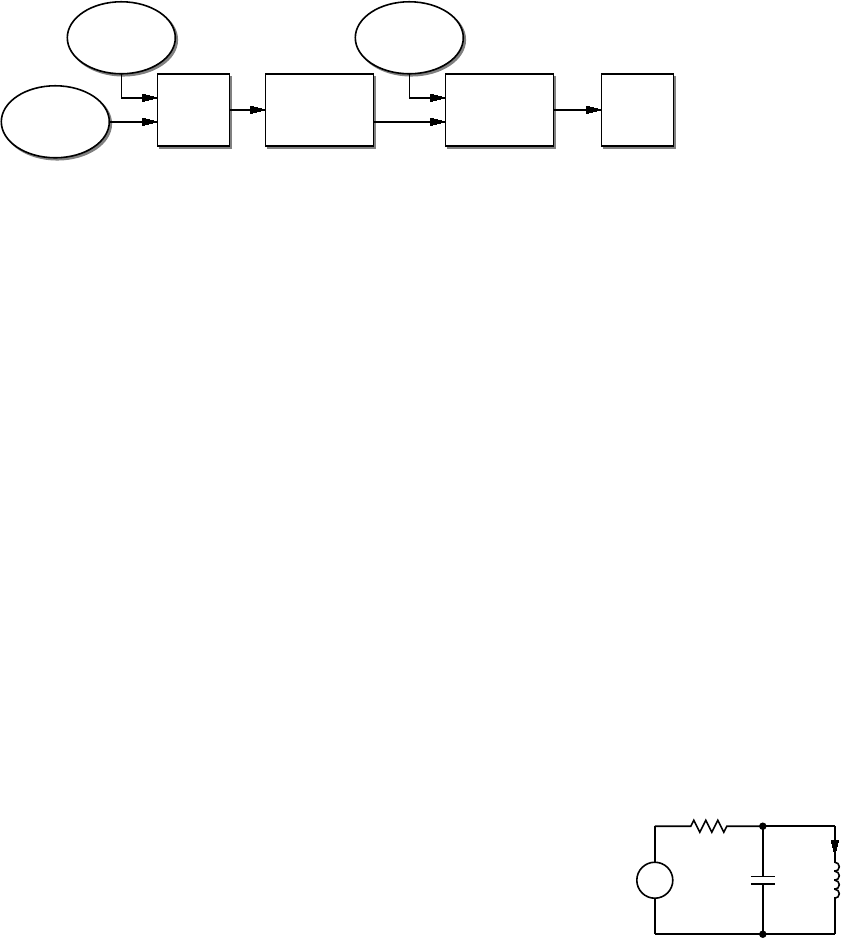2 Using the macros
This section describes the basic process of adding circuit diagrams to L
A
T
E
X documents to produce
postscript or pdf ﬁles. On some operating systems, project management software with graphical
interfaces can automate the process, but the steps can also be performed by a script, makeﬁle, or by
hand for simple documents as described in Section 2.1.
The diagram source ﬁle is preprocessed as illustrated in Figure 1. A conﬁguration ﬁle is read by
m4, followed by the diagram source. The result is passed through a pic interpreter to produce
.tex
output that can be inserted into a .tex document using the \input command.
.m4
diagram
.m4
macros
m4
pic
interpreter
.tex
ﬁles
L
A
T
E
X
or
PDFlatex
.dvi
or
.pdf
Figure 1: Inclusion of ﬁgures and macros in the L
A
T
E
X document.
The interpreter output contains Tikz PGF [
16
] commands, PSTricks [
17
] commands, basic L
A
T
E
X
graphics, tpic specials, or other formats, depending on the chosen options. These variations are
described in Section 15.
There are two principal choices of pic interpreter. One is dpic, described later in this document.
A partial alternative is GNU gpic -t (sometimes simply named pic) [
11
] together with a printer driver
that understands tpic specials, typically dvips [
14
]. The dpic processor extends the pic language in
small but important ways; consequently, some of the macros and examples in this distribution work
fully only with dpic. Pic processors contain basic macro facilities, so some of the concepts applied
here do not require m4.
2.1 Quick start
The contents of ﬁle
quick.m4
and resulting diagram are shown in Figure 2 to illustrate the language
and the production of basic labeled circuits.
.PS # Pic input begins with .PS
cct_init # Read in macro definitions and set defaults
elen = 0.75 # Variables are allowed; default units are inches
Origin: Here # Position names are capitalized
source(up_ elen); llabel(-,v_s,+)
resistor(right_ elen); rlabel(,R,)
dot
{ # Save the current position and direction
capacitor(down_ to (Here,Origin)) #(Here,Origin) = (Here.x,Origin.y)
rlabel(+,v,-); llabel(,C,)
dot
} # Restore position and direction
line right_ elen*2/3
inductor(down_ Here.y-Origin.y); rlabel(,L,); b_current(i)
line to Origin
.PE # Pic input ends
v
s
+
R
+
v
C L
i
Figure 2:
The ﬁle
quick.m4
and resulting diagram. There are several ways of drawing the same picture;
for example, nodes (such as
Origin
) can be deﬁned and circuit branches drawn between them; or
absolute coordinates can be used (e.g.,
source(up_ from (0,0) to (0,0.75))
). Element sizes
and styles can be varied as described in later sections.
2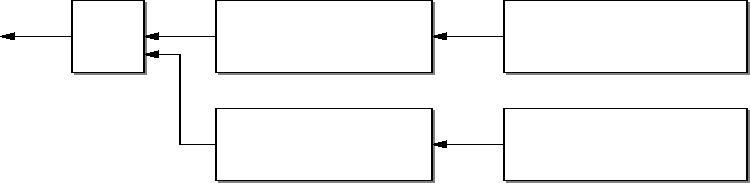2.1.1 Using m4
The command
m4 ﬁlename . . .
causes m4 to search for the named ﬁles in the current directory and directories speciﬁed by
environmental variable
M4PATH
. Set
M4PATH
to the full name (i.e., the path) of the directory
containing
libcct.m4
and the other circuit library
.m4
ﬁles; otherwise invoke m4 as
m4 -I
installdir
where installdir is the path to the directory containing the library ﬁles. Now there are at least two
basic possibilities as follows, but be sure to read Section 2.1.4 for simpliﬁed use.
2.1.2 Processing with dpic and PSTricks or Tikz PGF
If you are using dpic with PSTricks, put
\usepackage{pstricks}
in the main L
A
T
E
X source ﬁle
header and type the following commands or put them into a script:
m4 pstricks.m4 quick.m4 > quick.pic
dpic -p quick.pic > quick.tex
To produce Tikz PGF code, the L
A
T
E
\usepackage{tikz}
. The commands
are modiﬁed to read pgf.m4 and invoke the -g option of dpic as follows:
m4 pgf.m4 quick.m4 > quick.pic
dpic -g quick.pic > quick.tex
A conﬁguration ﬁle (
pstricks.m4
and
pgf.m4
in the above examples) is always the ﬁrst ﬁle to
be given to m4. Put the following or its equivalent in the document body:
\begin{figure}[ht]
\centering
\input quick
\caption{Customized caption for the figure.}
\label{Symbolic_label}
\end{figure}
Then for PSTricks, the commands
latex
ﬁle
; dvips
ﬁle produce ﬁle
.ps
, which can be printed or
viewed using
gsview
, for example. For Tikz PGF, Invoking PDFlatex on the source produces
.pdf
output directly. The essential line is \input quick whether or not the ﬁgure environment is used.
The eﬀect of the m4 command above is shown in Figure 3. Conﬁguration ﬁles
pstricks.m4
or
pgf.m4
cause library
libgen.m4
to be read, thereby deﬁning the macro
cct_init
. The diagram
source ﬁle is then read and the circuit-element macros in
libcct.m4
are deﬁned during expansion
of cct_init.
.pic
m4
Conﬁguration ﬁle
pstricks.m4 libgen.m4
· · ·
define(‘cct_init’,...)
· · ·
Diagram source quick.m4
.PS
cct_init
· · ·
libcct.m4
· · ·
define(‘resistor’,...)
· · ·
Figure 3: The command m4 pstricks.m4 quick.m4 > quick.pic.
2.1.3 Processing with gpic
If your printer driver understands tpic specials and you are using gpic (on some systems the gpic
command is pic), the commands are
3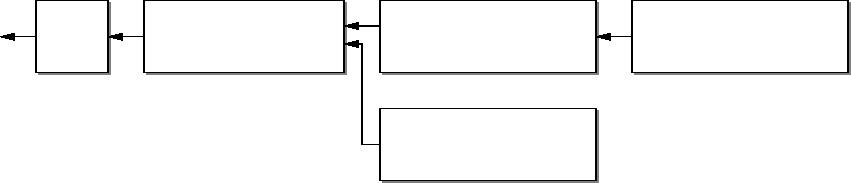m4 gpic.m4 quick.m4 > quick.pic
gpic -t quick.pic > quick.tex
and the ﬁgure inclusion statements are as shown:
\begin{figure}[ht]
\input quick
\centerline{\box\graph}
\caption{Customized caption for the figure.}
\label{Symbolic_label}
\end{figure}
2.1.4 Simpliﬁcations
M4 must read a conﬁguration ﬁle before any other ﬁles, either before reading the diagram source ﬁle
or at the beginning of it. There are several ways to control the process, as follows:
1.
The macros can be processed by L
A
T
E
X-speciﬁc project software and by graphic applications
such as Pycirkuit [
12
]. Alternatively when many ﬁles are to be processed, a facility such as
Unix “make,” which is also available in PC and Mac versions, can be employed to automate
the required commands. On systems without such facilities, a scripting language can be used.
2. The m4 commands illustrated above can be shortened to
m4 quick.m4 > quick.pic
by inserting
include(pstricks.m4)
(assuming PSTricks processing) immediately after the
.PS
line, the eﬀect of which is shown in Figure 4. However, if you then want to use Tikz PGF,
the line must be changed to include(pgf.m4).
.pic
m4
Diagram source
.PS
include(pstricks.m4)
cct_init
· · ·
Conﬁguration ﬁle
pstricks.m4 libgen.m4
· · ·
define(‘cct_init’,...)
· · ·
libcct.m4
· · ·
define(‘resistor’,...)
· · ·
Figure 4:
The command
m4 quick.m4 > quick.pic
, with
include(pstricks.m4)
preceding
cct_init
.
3.
In the absence of a need to examine the ﬁle
quick.pic
, the commands for producing the
.tex
ﬁle can be reduced (provided the above inclusions have been made) to
m4 quick.m4 | dpic -p > quick.tex
4.
You can put several diagrams into a single source ﬁle. Make each diagram the body of a L
A
T
E
X
macro, as shown:
\newcommand{\diaA}{%
.PS
drawing commands
.PE
\box\graph }% \box\graph not required for dpic
\newcommand{\diaB}{%
.PS
drawing commands
.PE
\box\graph }% \box\graph not required for dpic
Produce a
.tex
ﬁle as usual, insert the
.tex
into the L
A
T
E
X source, and invoke the macros
\diaA and \diaB at the appropriate places.
4
5.
In some circumstances, it may be desirable to invoke m4 and dpic automatically from the
document. Deﬁne a macro \mtotex as shown in the following example:
\documentclass{article}
\usepackage{tikz}
\newcommand\mtotex{\immediate\write18{m4 #2.m4 | dpic -#1 > #2.tex}}%
\begin{document}
\mtotex{g}{FileA} % Generate FileA.tex
\input{FileA.tex} \par
\mtotex{g}{FileB} % Generate FileB.tex
\input{FileB.tex}
\end{document}
The ﬁrst argument of
\mtotex
is a
p
for pstricks or
g
for pgf. Sources
FileA.m4
and
FileB.m4
must contain any required
include
statements, and the main document should be processed
using the latex or pdﬂatex option
--shell-escape
. If the
M4PATH
environment variable is
not set then insert
-I
installdir after
m4
in the command deﬁnition, where installdir is the
absolute path to the installation directory. This method processes the picture source each
time L
A
T
E
X is run, so for large documents containing many diagrams, the
\mtotex
lines could
be commented out after debugging the corresponding graphic. A derivative of this method
that allows the insertion of pic code into a Tikz picture is described in Section 14.1.
6.
It might be convenient for the source of small diagrams to be part of the document source text.
The
filecontents
environment of current L
A
T
E
X allows this; older versions can employ a
now-obsolete package
filecontents.sty
. The following example for processing by
pdflatex
--shell-escape
ﬁrst writes the m4 source to ﬁle
sample.m4
, invokes
\mtotex
in the result:
include(pgf.m4)
.PS
cct_init
drawing commands . . .
.PE
\end{filecontents}
\mtotex{g}{sample}
\input{sample.tex}
2.2 Including the libraries
The conﬁguration ﬁles for dpic are as follows, depending on the output format (see Section 15):
pstricks.m4, pgf.m4, mfpic.m4, mpost.m4, postscript.m4, psfrag.m4, svg.m4, gpic.m4,
or
xfig.m4
. The ﬁle
psfrag.m4
simply deﬁnes the macro
psfrag_
postscript.m4
.
For gpic, the conﬁguration ﬁle is
gpic.m4
. The usual case for producing circuit diagrams is to read
pstricks.m4
or
pgf.m4
ﬁrst when dpic is the postprocessor or to set one of these as the default
conﬁguration ﬁle.
At the top of each diagram source, put one or more initialization commands; that is,
cct_init, log_init, sfg_init, darrow_init, threeD_init
or, for diagrams not requiring specialized macros,
gen_init
. As shown in Figures 3 and 4, each
example, cct_init tests whether libcct.m4 has been read and includes it if necessary.
A few of the distributed example ﬁles contain other experimental macros that can be pasted into
diagram source ﬁles; see Flow.m4 or Buttons.m4, for example.
The libraries contain hints and explanations that might help in debugging or if you wish to
modify any of the macros. Macros are generally named using the obvious circuit element names
so that programming becomes something of an extension of the pic language. Some macro names
5
end in an underscore to reduce the chance of name clashes. These can be invoked in the diagram
source but there is no long-term guarantee that their names and functionality will remain unchanged.
Finally, macros intended only for internal use begin with the characters m4.
3 Pic essentials
Pic source is a sequence of lines in a text ﬁle. The ﬁrst line of a diagram begins with
.PS
with
optional following arguments, and the last line is normally
.PE
. Lines outside of these pass through
the pic processor unchanged.
The visible objects can be divided conveniently into two classes, the linear objects
line, arrow,
spline, arc, and the planar objects box, circle, ellipse.
The object
move
is linear but draws nothing. A compound object, or
block,
is planar and
consists of a pair of square brackets enclosing other objects, as described in Section 3.5.
Objects can be placed using absolute coordinates or, as is often better, relative to other objects.
Pic allows the deﬁnition of real-valued variables, which are alphameric names beginning with
lower-case letters, and computations using them. Objects or locations on the diagram can be given
symbolic names beginning with an upper-case letter.
3.1 Manuals
The classic pic manual [
8
] is still a good introduction to pic, but a more complete manual [
13
] can be
found in the GNU groﬀ package, and both are available on the web [
8
,
13
you competence with pic in an hour or two. Explicit mention of
*roff
string and font constructs in
these manuals should be replaced by their equivalents in the L
A
T
E
X context. A man-page language
summary is appended to the dpic manual .
A web search will yield good discussions of “little languages”; for pic in particular, see Chapter 9
of . Chapter 1 of reference  also contains a brief discussion of this and other languages.
3.2 The linear objects: line, arrow, spline, arc
A line can be drawn as follows:
line from position to position
where position is deﬁned below or
line direction distance
where direction is one of
up, down, left, right.
When used with the m4 macros described here,
it is preferable to add an underscore:
up_, down_, left_, right_.
The distance is a number or
expression and the units are inches, but the assignment
scale = 25.4
has the eﬀect of changing the units to millimetres, as described in Section 11.
Lines can also be drawn to any distance in any direction. The example,
line up_ 3/sqrt(2) right_ 3/sqrt(2) dashed
draws a line 3 units long from the current location, at a 45
angle above horizontal. Lines (and
other objects) can be speciﬁed as dotted, dashed, or invisible, as above.
The construction
line from A to B chop x
truncates the line at each end by
x
(which may be negative) or, if
x
is omitted, by the current circle
radius, a convenience when A and B are circular graph nodes, for example. Otherwise
line from A to B chop x chop y
truncates the line by x at the start and y at the end.
Any of the above means of specifying line (or arrow) direction and length will be called a linespec.
Lines can be concatenated. For example, to draw a triangle:
line up_ sqrt(3) right_ 1 then down_ sqrt(3) right_ 1 then left_ 2
6
3.3 Positions
A position can be deﬁned by a coordinate pair, e.g.
3,2.5
, more generally using parentheses by
(
expression, expression
)
, as a sum or diﬀerence as position +
(
expression, expression
)
, or by the
construction
(
position, position
)
, the latter taking the
x
-coordinate from the ﬁrst position and
the
y
-coordinate from the second. A position can be given a symbolic name beginning with an
upper-case letter, e.g.
Top: (0.5,4.5)
. Such a deﬁnition does not aﬀect the calculated ﬁgure
boundaries. The current position
Here
is always deﬁned and is equal to (0
,
0) at the beginning of a
diagram or block. The coordinates of a position are accessible, e.g.
Top.x
and
Top.y
can be used in
expressions. The center, start, and end of linear objects (and the deﬁned points of other objects as
described below) are predeﬁned positions, as shown in the following example, which also illustrates
how to refer to a previously drawn element if it has not been given a name:
line from last line.start to 2nd last arrow.end then to 3rd line.center
Objects can be named (using a name commencing with an upper-case letter), for example:
Bus23: line up right
after which, positions associated with the object can be referenced using the name; for example:
arc cw from Bus23.start to Bus23.end with .center at Bus23.center
An arc is drawn by specifying its rotation, starting point, end point, and center, but sensible
defaults are assumed if any of these are omitted. Note that
arc cw from Bus23.start to Bus23.end
does not deﬁne the arc uniquely; there are two arcs that satisfy this speciﬁcation. This distribution
includes the m4 macros
arcd( position, radius, start degrees, end degrees, modiﬁers, ht)
arca( chord linespec, ccw|cw, radius, modiﬁers)
to draw uniquely deﬁned arcs. If the ﬁfth argument of
arcr
or
arcd
contains
->
or
<-
then a
arcd((1,-1),,0,-90,<- outlined "red") dotted
draws a red dotted arc with midpoint arrowhead, centre at (1
,
1)
,
arca(from (1,1) to (2,2),,1,->)
draws an acute angled arc with arrowhead on the chord deﬁned by the ﬁrst argument.
The linear objects can be given arrowheads at the start, end, or both ends, for example:
line dashed <- right 0.5
arc <-> height 0.06 width 0.03 ccw from Here to Here+(0.5,0) \
with .center at Here+(0.25,0)
spline -> right 0.5 then down 0.2 left 0.3 then right 0.4
height
and
width
parameters.
3.4 The planar objects: box, circle, ellipse, and text
Planar objects are drawn by specifying the width, height, and position, thus:
A: box ht 0.6 wid 0.8 at (1,1)
after which, in this example, the position
A.center
is deﬁned, and can be referenced simply as
A
.
The compass points
A.n, A.s, A.e, A.w, A.ne, A.se, A.sw, A.nw
are automatically deﬁned, as
are the dimensions
A.height
and
A.width.
Planar objects can also be placed by specifying the
location of a deﬁned point; for example, two touching circles can be drawn as shown:
circle diameter (last circle.width * 1.2) with .sw at last circle.ne
The planar objects can be ﬁlled with gray or colour. For example, either
box dashed fill_(number) or box dashed outlined "color" shaded "color"
produces a dashed box. The ﬁrst case has a gray ﬁll determined by number, with 0 corresponding
to black and 1 to white; the second case allows color outline and ﬁll, the color strings depending
on the postprocessor. Postprocessor-compatible RGB color strings are produced by the macro
rgbstring(red fraction, green fraction, blue fraction); to produce an orange ﬁll for example:
... shaded rgbstring( 1, 0.645, 0)
7
Basic colours for lines and ﬁlls are provided by gpic and dpic, but more elaborate line and ﬁll
styles or other eﬀects can be incorporated, depending on the postprocessor, using
command "string"
where string is one or more postprocessor command lines.
Arbitrary text strings, typically meant to be typeset by L
A
T
E
X, are delimited by double-quote
characters and occur in two ways. The ﬁrst way is illustrated by
"\large Resonances of $C_{20}H_{42}$" wid x ht y at position
which writes the typeset result, like a box, at position and tells pic its size. The default size assumed
by pic is given by parameters
textwid
and
textht
if it is not speciﬁed as above. The exact typeset
size of formatted text can be obtained as described in Section 13. The second occurrence associates
one or more strings with an object, e.g., the following writes two words, one above the other, at the
centre of an ellipse:
ellipse "\bf Stop" "\bf here"
The C-like pic function sprintf("format string",numerical arguments) is equivalent to a string.
3.5 Compound objects
A compound object is a group of statements enclosed in square brackets. Such an object is placed
by default as if it were a box, but it can also be placed by specifying the ﬁnal position of a deﬁned
point. A deﬁned point is the center or compass corner of the bounding box of the compound object
or one of its internal objects. Consider the last line of the code fragment shown:
Ands: [ right_
And1: AND_gate
And2: AND_gate at And1 - (0,And1.ht*3/2)
. . .
] with .And2.In1 at position
The two gate macros evaluate to compound objects containing
Out
,
In1
, and other locations. The
ﬁnal positions of all objects inside the square brackets are determined in the last line by specifying
the position of In1 of gate And2.
3.6 Other language facilities
All objects have default sizes, directions, and other characteristics, so part of the speciﬁcation of an
object can sometimes be proﬁtably omitted.
Another possibility for deﬁning positions is
expression between position and position
which means
1st position + expression × (2nd position 1st position)
and which can be abbreviated as
expression < position , position >
Care has to be used in processing the latter construction with m4, since the comma may have to be
put within quotes, ‘,’ to distinguish it from the m4 argument separator.
Positions can be calculated using expressions containing variables. The scope of a position is the
current block. Thus, for example,
theta = atan2(B.y-A.y,B.x-A.x)
line to Here+(3*cos(theta),3*sin(theta)).
Expressions are the usual algebraic combinations of primary quantities: constants, environmental
parameters such as
scale,
variables, horizontal or vertical coordinates of terms such as position
.x
or position
.y
, dimensions of pic objects, e.g.
. The elementary algebraic operators
are +, -, *, /, %, =, +=, -=, *=, /=, and %=, similar to the C language.
The logical operators
==, !=, <=, >=, >,
and
<
apply to expressions and strings. A modest
selection of numerical functions is also provided: the single-argument functions
sin, cos, log,
exp, sqrt, int
, where
log
and
exp
are base-10, the two-argument functions
atan2, max, min,
and the random-number generator rand(). Other functions are also provided using macros.
8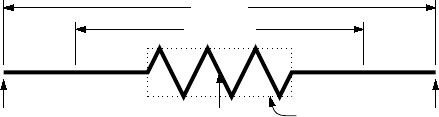A pic manual should be consulted for details, more examples, and other facilities, such as the
branching facility
if expression then { anything } else { anything },
the looping facility
for variable = expression to expression by expression do { anything },
operating-system commands, pic macros, and external ﬁle inclusion.
4 Two-terminal circuit elements
There is a fundamental diﬀerence between the two-terminal elements, each of which is drawn along
an invisible straight-line segment, and other elements, which are compound objects mentioned in
Section 3.5. The two-terminal element macros follow a set of conventions described in this section,
and other elements will be described in Section 6.
4.1 Circuit and element basics
A list of the library macros and their arguments is in Section 18. The arguments have default values,
so that only those that diﬀer from defaults need be speciﬁed.
Figure 5, which shows a resistor, also serves as an example of pic commands. The ﬁrst part of
the source ﬁle for this ﬁgure is on the left:
.PS
cct_init
linewid = 2.0
linethick_(2.0)
R1: resistor
last []
R1.start R1.endR1.centre
elen_
dimen_
Figure 5: Resistor named R1, showing the size parameters, enclosing block, and predeﬁned positions.
The lines of Figure 5 and the remaining source lines of the ﬁle are explained below:
The ﬁrst line invokes the macro
cct_init
libcct.m4
and initializes
local variables needed by some circuit-element macros.
The sizes of circuit elements are proportional to the pic environmental variable
linewid
, so
redeﬁning this variable changes element sizes. The element body is drawn in proportion to
dimen_
, a macro that evaluates to
linewid
unless redeﬁned, and the default element length
is
elen_
, which evaluates to
dimen_*3/2
unless redeﬁned. Setting
linewid
to 2.0 as in the
example means that the default element length becomes 3.0 in. For resistors, the default length
of the body is
dimen_/2,
and the width is
dimen_/6.
All of these values can be customized.
Element scaling and the use of SI units is discussed further in Section 11.
The macro
linethick_
sets the default thickness of subsequent lines (to 2.0 pt in the example).
Macro arguments are written within parentheses following the macro name, with no space
between the name and the opening parenthesis. Lines can be broken before macro arguments
because m4 and dpic ignore white space immediately preceding arguments. Otherwise, a long
line can be continued to the next by putting a backslash as the rightmost character.
The two-terminal element macros expand to sequences of drawing commands that begin with
‘line invis
linespec
, where linespec is the ﬁrst argument of the macro if it is non-blank,
otherwise the line is drawn a distance
elen_
in the current direction, which is to the right
by default. The invisible line is ﬁrst drawn, then the element is drawn on top of it. The
element—rather, the initial invisible line—can be given a name,
R1
in the example, so that
positions R1.start, R1.centre, and R1.end are automatically deﬁned as shown.
9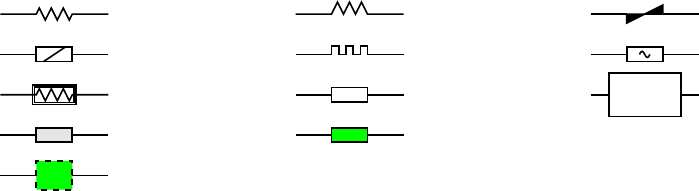The element body is overlaid by a block, which can be used to place labels around the element.
The block corresponds to an invisible rectangle with horizontal top and bottom lines, regardless
of the direction in which the element is drawn. A dotted box has been drawn in the diagram
to show the block boundaries.
The last sub-element, identical to the ﬁrst in two-terminal elements, is an invisible line that
can be referenced later to place labels or other elements. If you create your own macros, you
might choose simplicity over generality, and include only visible lines.
To produce Figure 5, the following embellishments were added after the previously shown source:
thinlines_
box dotted wid last [].wid ht last [].ht at last []
move to 0.85 between last [].sw and last [].se
spline <- down arrowht*2 right arrowht/2 then right 0.15; "\tt last []" ljust
arrow <- down 0.3 from R1.start chop 0.05; "\tt R1.start" below
arrow <- down 0.3 from R1.end chop 0.05; "\tt R1.end" below
arrow <- down last [].c.y-last arrow.end.y from R1.c; "\tt R1.centre" below
dimension_(from R1.start to R1.end,0.45,\tt elen\_,0.4)
dimension_(right_ dimen_ from R1.c-(dimen_/2,0),0.3,\tt dimen\_,0.5)
.PE
The line thickness is set to the default thin value of
0.4 pt
, and the box displaying the element
body block is drawn. Notice how the width and height can be speciﬁed, and the box centre
positioned at the centre of the block.
The next paragraph draws two objects, a spline with an arrowhead, and a string left justiﬁed
at the end of the spline. Other string-positioning modiﬁers than
ljust
are
rjust, above,
and below.
The last paragraph invokes a macro for dimensioning diagrams.
4.2 The two-terminal elements
The two-terminal elements are shown in Figures 6 to 15 and part of Figure 16. Several elements
are included more than once to illustrate some of their arguments, which are listed in detail in
Section 18. Figure 6 shows some resistors with typical variants.
resistor
resistor(,,Q) resistor(,,V)
resistor(,,ES) resistor(,,H) resistor(,,AC)
resistor(,,B)
resistor(,,E)
ebox
ebox(,0.5,0.3)
ebox(,,,0.9)
X
Figure 6:
Resistors dawn by the macro
resistor(
linespec, n
|E,
chars
,
cycle wid
)
. The second argument
is either an integer to specify number of cycles, the letter
E
, or blank. The third argument speciﬁes
the desired variant. The default ebox element designates a resistor.
The ﬁrst macro argument speciﬁes the invisible line segment along which the element is drawn.
If the argument is blank, the element is drawn from the current position in the current drawing
direction along a default length. The other arguments produce variants of the default elements.
Thus, for example,
resistor(up_ 1.25,7)
draws a resistor 1.25 units long up from the current position, with 7 vertices per side. The macro
up_ evaluates to up but also resets the current directional parameters to point up.
10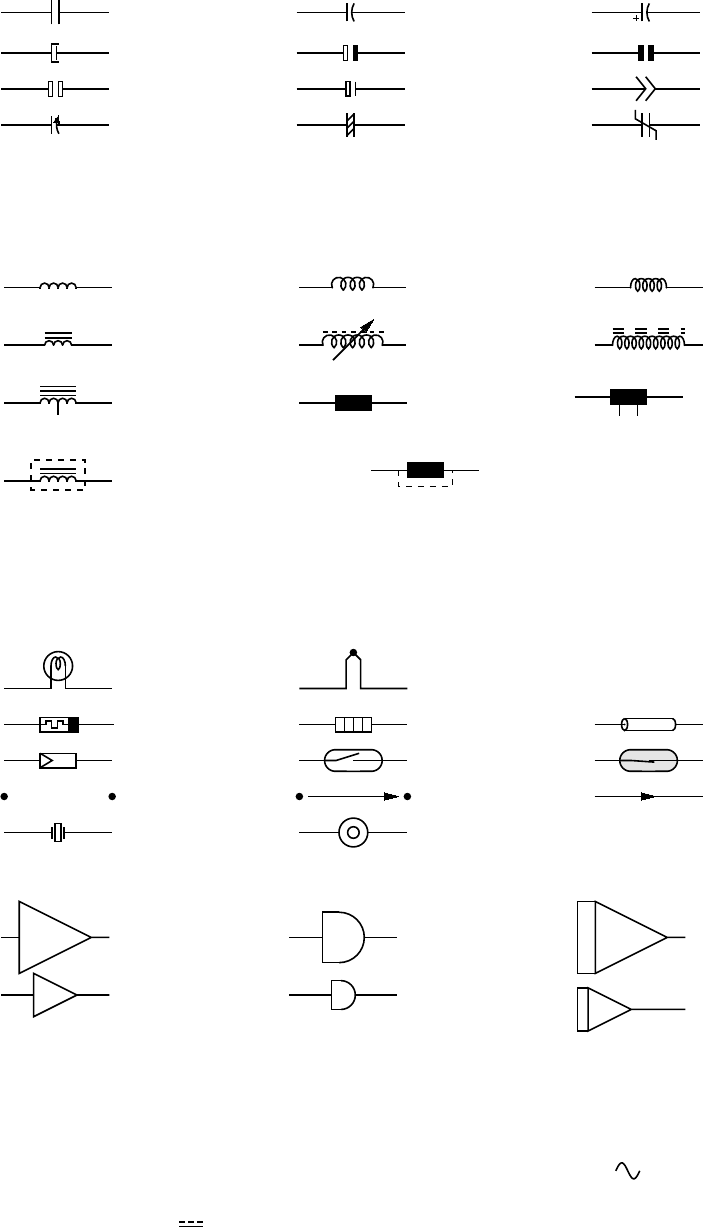Capacitors are illustrated in Figure 7. See Section 6 for the variable macro.
capacitor
capacitor(,C) capacitor(,C+)
capacitor(,P) capacitor(,E) capacitor(,K)
capacitor(,M) capacitor(,N) capacitor(,CP)
capacitor(,dC) capacitor(,dF)
variable(capacitor’,
NN,-30,dimen_/3)
Figure 7:
The
capacitor(
linespec, chars,
[R],
height, width
)
macro, and an example application of the
variable macro.
Basic inductors are illustrated in Figure 8.
inductor
inductor(,W) inductor(,L)
...(„3,M)
...(,W,6,P1);
variable
...(,L,9,K)
...(,,,M3)
ebox(,,,0)
tapped(‘ebox(,,,0)’,
-,1/4,-dimen_/6,
3/4,-dimen_/6)
Tap1 Tap2
shielded(
‘inductor(,,,M)’)
shielded(‘ebox(,,,0)’,R)
Figure 8:
Basic inductors created with the
inductor(
linespec,
W|L,
cycles,
M|P|K,
loop wid
)
macro,
the
ebox
macro for European-style inductors, and some modiﬁcations (see also Section 6). When an
embellished element is repeated several times, writing a wrapper macro may be desirable.
Some more basic elements are in Figure 9, and ampliﬁers in Figure 10.
lamp thermocouple
memristor heater tline
pvcell
reed
reed(,,,fill_(0.9),CR)
gap
gap(,,A)
arrowline
xtal
xtal(,type=R)
Figure 9: More two-terminal elements.
amp
amp(,0.3)
delay
delay(,0.2)
integrator
integrator(,0.3)
Figure 10: Ampliﬁer, delay, and integrator.
Figure 11 shows sources, many of which contain internal symbols, and of which the
AC
and
S
options illustrate the need to draw a single cycle of a sinusoid or approximate sinusoid. As a
convenience, the macro
ACsymbol(at
position, length, height,
[A]U|D|L|R|
degrees
)
is included as
an interface to the sinusoid macro. For example to add the sumbol to an ebox:
ebox; { ACsymbol(at last [],,,dimen_/8) }
For direct current ( ), there is also
DCsymbol(at
position, length, height,
U|D|L|R|
degrees
)
,
and for power-system diagrams, macros
Deltasymbol(at
position, keys,
U|D|L|R|
degrees
)
, and
Ysymbol(at position, keys, U|D|L|R|degrees),
11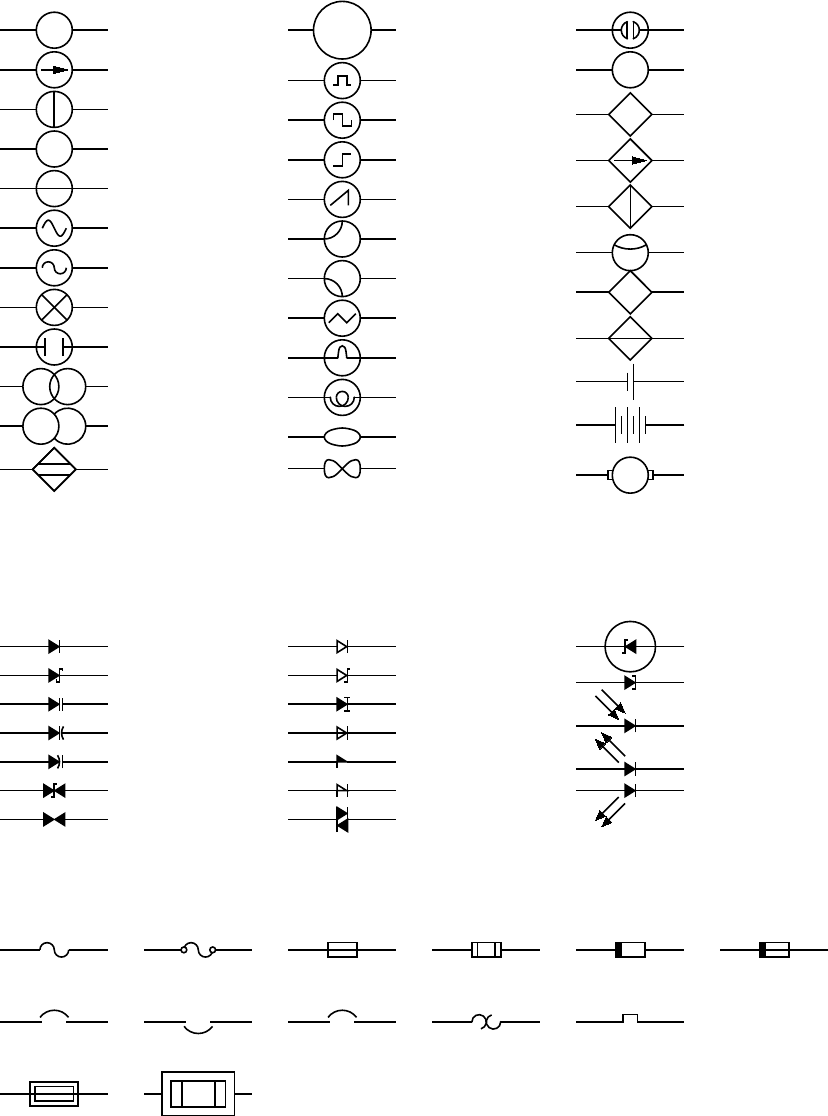source
source(,I)
source(,i)
+
source(,V)
source(,v)
source(,S)
source(,AC)
source(,X)
source(,F)
source(,G)
source(,Q)
proximity
= consource(,P)
source(,,0.4)
source(,P)
source(,U)
source(,H)
source(,R)
source(,SC)
source(,SCr)
source(,T)
source(,L)
source(,B)
nullator
norator
source(,N)
mA
source(,"mA")
consource
consource(,I)
consource(,i)
source(,SE)
+
consource(,V)
consource(,v)
battery
battery(,3,R)
G
ttmotor(,G)
Figure 11: Sources and source-like elements.
Diodes and fuses are shown in Figures 12 and 13.
diode
diode(,S)
diode(,V)
diode(,v)
diode(,w)
diode(,B)
diode(,G)
diode(,K)
diode(,ZK)
diode(,CR)
diode(,L)
diode(,F)
diode(,Sh)
diode(,D)
diode(,Z,RE)
diode(,T)
diode(,P)
diode(,LE)
diode(,LER)
Figure 12:
The macro
diode(
linespec
,B|CR|D|L|LE[R]|P[R]|S|T|V|v|w|Z|
chars
,[R][E])
. Appending
K to the second argument draws an open arrowhead.
fuse
fuse(,D) fuse(,B) fuse(,C) fuse(,S) fuse(,SB)
cbreaker
cbreaker(,R) . . .(,,D) . . .(,,T) . . .(,,TS)
fuse(,HB)
(,HC,0.5,0.3)
Figure 13:
Variations of the macros
fuse(
linespec
, A|dA|B|C|D|E|S|HB|HC|SB,
wid
,
ht
)
and
cbreaker(linespec,L|R,D|T|TS).
Most of the two-terminal elements are oriented; that is, they have a deﬁned direction or polarity.
Several element macros include an argument that reverses polarity, but there is also a more general
mechanism, as follows.
The ﬁrst argument of the macro
reversed(‘macro name’,macro arguments)
12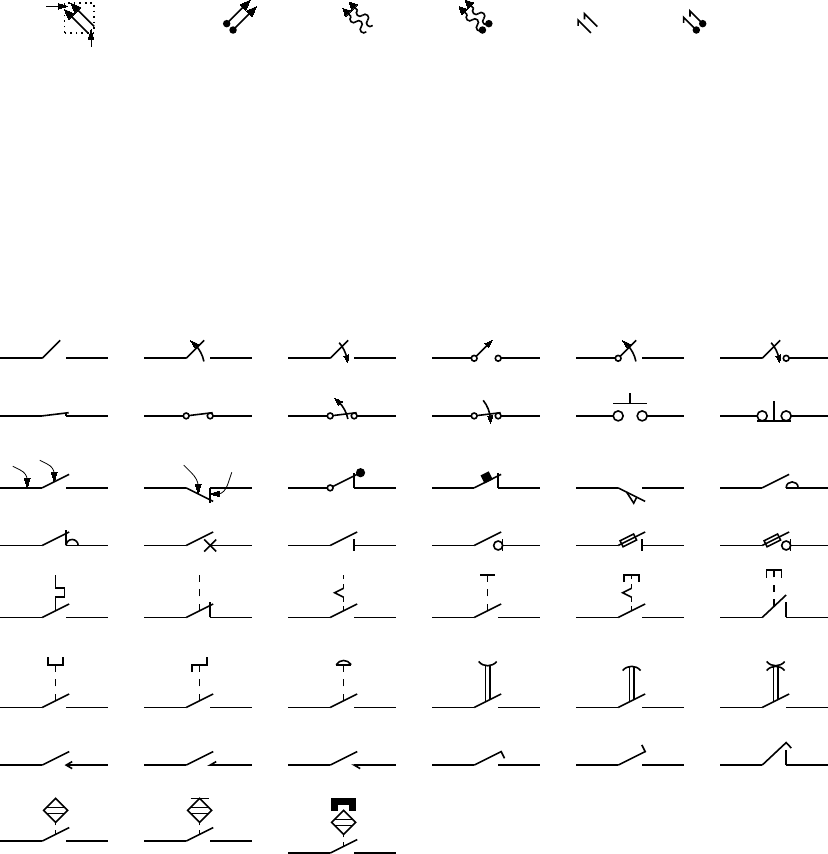is the name of a two-terminal element in quotes, followed by the element arguments. The element is
drawn with reversed direction; thus,
diode(right_ 0.4); reversed(‘diode’,right_ 0.4)
draws two diodes to the right, but the second one points left.
Similarly, the macro
resized(factor,‘macro name’,macro arguments)
can be used to resize the body of an element by temporarily multiplying the
dimen_
macro by factor.
More general resizing should be done by redeﬁning
dimen_
as described in Section 11.1. These two
macros can be nested; the following scales the above example by 1.8, for example
resized(1.8,‘diode’,right_ 0.4); resized(1.8,‘reversed’,‘diode’,right_ 0.4)
Figure 14 contains radiation-eﬀect arrows for embellishing two-terminal and other macros. The
Tail
A1
A2
em_arrows(N)
em_arrows(ND,45) . . .(I) . . .(ID) . . .(E) . . .(ED)
Figure 14: Radiation arrows: em_arrows(type, angle, length)
arrow stems are named A1, A2, and each pair is drawn in a
[]
block, with the names Head and
Tail deﬁned to aid placement near another device. The second argument speciﬁes absolute angle in
degrees (default 135 degrees). The arrows are drawn relative to the diode direction by the
LE
option
in Figure 12. For absolute arrow directions, one can deﬁne a wrapper (see Section 12) for the
diode
macro to draw arrows at 45 degrees, for example:
define(‘myLED’,‘diode(‘$1’); em_arrows(N,45) with .Tail at last [].ne’) Switches with numerous controls are in Figure 15. lswitch (,,O) (,,C) (,,DA) (,,dDO) (,,uDC) (,,K) (,,KD) (,,KOD) (,,KCD) bswitch (,,C) dswitch(,,) W B (,,WdBK) dB K (,,WBmdDK) (,,WBKTr) (,,WdBL) (,,WBCo) (,,WBKCo) (,,WBCb) (,,WBDI) (,,WBSd) (,,WBFDI) (,,WBFSd) (,,WBTh) (,,WBKC) (,,WBM) (,,WBCO) (,,WBMP) (,,WBoKCP) (,,WBCY) (,,WBCZ) (,,WBCE) (,,WBRH) (,,WBRdH) (,,WBRHH) (,,WBMMR) (,,WBMM) (,,WBMR) (,,WBEL) (,,WBLE) (,,WBoKEL) (,,WBPr) (,,WBPrT) (,,WBPrM) Figure 15: The switch( linespec ,L|R, chars ,L|B|D) macro is a wrapper for the macros lswitch( linespec ,[L|R],[O|C][D][K][A]) , bswitch( linespec ,[L|R],[O|C]) , and the many- optioned dswitch( linespec ,R,W[ud]B[K] chars ) shown. The switch is drawn in the current drawing direction. A second-argument R produces a mirror image with respect to the drawing direction. The separately deﬁned macros Proxim and Magn embellish switches in the second-last row. 13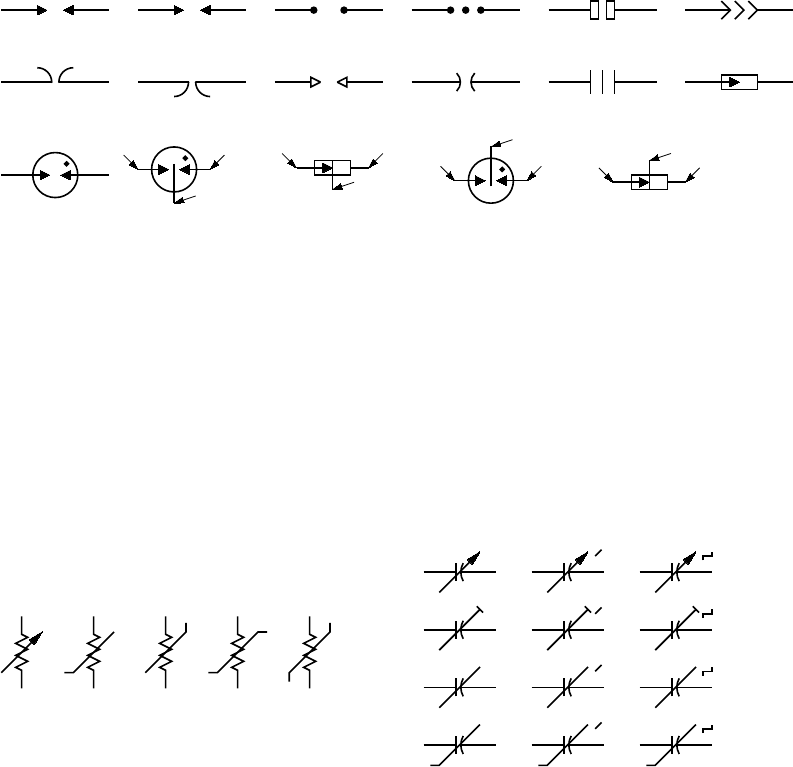Figure 16 shows a collection of surge-protection devices, or arresters, of which the E and S types may be either 2-terminal or as 3-terminal (composite) elements described in Section 6. arrester arrester(,G) ...(,g) ...(,M) ...(,C) ...(,A) ...(,H) ...(,HR) ...(,P) ...(,s) ...(,F) ...(,S) ...(,E) ...(,DE) A B G ...(,DS) A B G ...(,DEL) A B G ...(,DSL) A B G Figure 16: Variations of the arrester( linespec, chars, wid [: arrowhead ht ], ht [: arrowhead wid ]) macro. Putting D in argument 2 for the S or E conﬁguration creates a 3-terminal composite element with terminals A, B, and G. Figure 17 shows some two-terminal elements with arrows or lines overlaid to indicate variability using the macro variable(‘element’,type,[+|-]angle,length), where type is one of A, P, L, N, NN with C or S optionally appended to indicate continuous or stepwise variation. Alternatively, this macro can be invoked similarly to the label macros in Section 4.4 by specifying an empty ﬁrst argument; thus, the following line draws the third resistor in Figure 17: resistor(up_ dimen_); variable(,uN) A N uN NN uNN C S A P L N Figure 17: Illustrating variable(‘ element ’,[A|P|L|[u]N]|[u]NN]][C|S],[+|-] angle , length ) . For example, variable(‘resistor(up_ dimen_)’,A) draws the leftmost resistor shown above. The default angle is 45 , regardless of the direction of the element, but the angle preceded by a sign (+ or ) is taken to be relative to the drawing direction of the element as for the lower right capacitor in Figure 7, for example. The array on the right shows the eﬀect of the second argument. 4.3 Branch-current arrows Arrowheads and labels can be added to conductors using basic pic statements. For example, the following line adds a labeled arrowhead at a distance alpha along a horizontal line that has just been drawn. Many variations of this are possible: arrow right arrowht from last line.start+(alpha,0) "$i_1\$" above
Macros have been deﬁned to simplify labelling two-terminal elements, as shown in Figure 18.
The macro
b_current(label, above_|below_, In|O[ut], Start|E[nd], frac)
draws an arrow from the start of the last-drawn two-terminal element frac of the way toward the
body.
If the fourth argument is
End
, the arrow is drawn from the end toward the body. If the third
element is
Out
, the arrow is drawn outward from the body. The ﬁrst argument is the desired label,
of which the default position is the macro
above_,
which evaluates to
above
if the current direction
14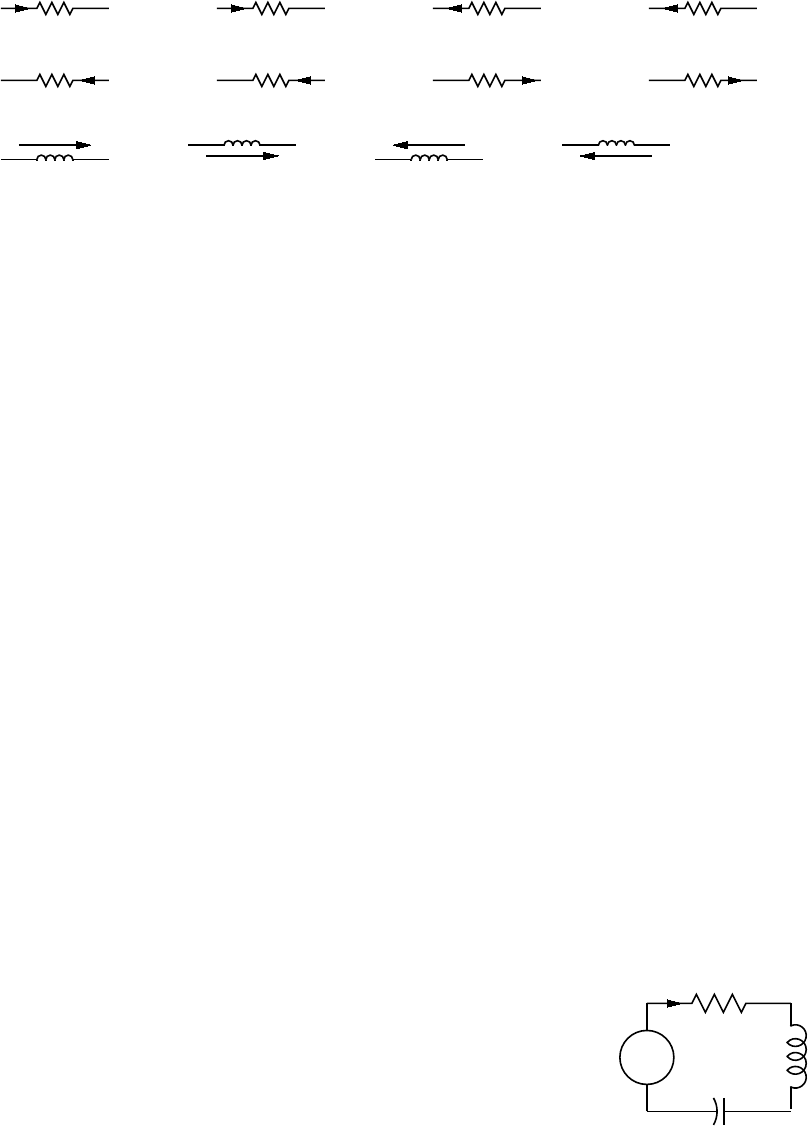i
b_current(i)
i
. . .(i,below_)
i
. . .(i,,O)
i
. . .(i,below_,O)
i
b_current(i,,,E)
i
. . .(i,below_,,E)
i
. . .(i,,O,E,0.2)
i
. . .(i,below_,O,E)
i
larrow(i)
i
rarrow(i)
i
larrow(i,<-)
i
rarrow(i,<-)
Figure 18: Illustrating b_current, larrow, and rarrow. The drawing direction is to the right.
is right or to
ljust, below, rjust
if the current direction is respectively down, left, up. The label
is assumed to be in math mode unless it begins with
sprintf
or a double quote, in which case it
is copied literally. A non-blank second argument speciﬁes the relative position of the label with
respect to the arrow, for example
below_,
which places the label below with respect to the current
direction. Absolute positions, for example below or ljust, also can be speciﬁed.
For those who prefer a separate arrow to indicate the reference direction for current, the macros
larrow(
label
, ->|<-,
dist
)
and
rarrow(
label
, ->|<-,
dist
)
are provided. The label is placed
outside the arrow as shown in Figure 18. The ﬁrst argument is assumed to be in math mode unless
it begins with
sprintf
or a double quote, in which case the argument is copied literally. The third
argument speciﬁes the separation from the element.
4.4 Labels
Arbitrary labels can be positioned by any pic placement method including the representative basic
examples shown:
"text" at position
"text" at position above
"text" wid width ht height with .sw at position
In addition, special macros for labeling two-terminal elements are available:
llabel( arg1,arg2,arg3 )
clabel( arg1,arg2,arg3 )
rlabel( arg1,arg2,arg3 )
dlabel( long,lat,arg1,arg2,arg3,[X][A|B][L|R])
The ﬁrst macro places the three arguments, which are treated as math-mode strings, on the
left side of the element block with respect to the current direction:
up, down, left, right.
The
second places the arguments along the centre, and the third along the right side. A simple circuit
example with labels is shown in Figure 19. The macro
dlabel
performs these functions for an
.PS
# ‘Loop.m4’
cct_init
define(‘dimen_’,0.75)
loopwid = 1; loopht = 0.75
source(up_ loopht); llabel(-,v_s,+)
resistor(right_ loopwid); llabel(,R,); b_current(i)
inductor(down_ loopht,W); rlabel(,L,)
capacitor(left_ loopwid,C); llabel(+,v_C,-); rlabel(,C,)
.PE
v
s
+
R
i
L
+
v
C
C
Figure 19: A loop containing labeled elements, with its source code.
obliquely drawn element, placing the three macro arguments at
vec_(-long,lat), vec_(0,lat),
and
vec_(long,lat)
respectively relative to the centre of the element. In the fourth argument, an
X
aligns the labels with respect to the line joining the two terminals rather than the element body,
15
and
A, B, L, R
use absolute
above, below, left,
or
right
alignment respectively for the labels.
Labels beginning with
sprintf
or a double quote are copied literally rather than assumed to be in
math mode.
5 Placing two-terminal elements
The length and position of a two-terminal element are deﬁned by a straight-line segment, so four
numbers or equivalent are required to place the element as in the following example:
resistor(from (1,1) to (2,1)).
However, pic has a very useful concept of the current point (explicitly named Here); thus,
resistor(to (2,1))
is equivalent to
resistor(from Here to (2,1)).
Any deﬁned position can be used; for example, if C1 and L2 are names of previously deﬁned
two-terminal elements, then, for example, the following places the resistor:
resistor(from L2.end to C1.start)
A line segment starting at the current position can also be deﬁned using a direction and length.
To draw a resistor up d units from the current position, for example:
resistor(up_ d)
Pic stores the current drawing direction, which is unfortunately limited to
up, down, left,
right,
for reference when necessary. The circuit macros need to know the current direction, so
whenever
up, down, left, right
are used they should be written respectively as the macros
up_,
down_, left_, right_ as in the above example.
To allow drawing circuit objects in other than the standard four directions, a transformation
matrix is applied at the macro level to generate the required (but sometimes very elaborate) pic
code. Potentially, the matrix elements can be used for other transformations. The macro
setdir_(direction, default direction)
is preferred when setting drawing direction. The direction arguments are of the form
R[ight] | L[eft] | U[p] | D[own] | degrees,
but the macros
Point_(
degrees
), point_(
),
and
rpoint_(
relative linespec
)
are employed
in many macros to re-deﬁne the entries of the matrix (named
m4a_
,
m4b_
,
m4c_
, and
m4d_
) for
the required rotation. The macro
eleminit_
in the two-terminal elements invokes
rpoint_
with a
speciﬁed or default linespec to establish element length and direction.
As shown in Figure 20,
Point_(-30); resistor
draws a resistor along a line with slope of -30
degrees, and
rpoint_(to Z)
sets the current direction cosines to point from the current location
to location Z. Macro
vec_(x,y)
evaluates to the position
(x,y)
rotated as deﬁned by the argument
of the previous
setdir_, Point_, point_
or
rpoint_
command. The principal device used to
deﬁne relative locations in the circuit macros is
rvec_(x,y)
, which evaluates to position
Here +
vec_(x,y). Thus, line to rvec_(x,0) draws a line of length x in the current direction.
Figure 20 illustrates that some hand placement of labels using
dlabel
may be useful when
elements are drawn obliquely. The ﬁgure also illustrates that any commas within m4 arguments
must be treated specially because the arguments are separated by commas. Argument commas are
protected either by parentheses as in
inductor(from Cr to Cr+vec_(elen_,0))
, or by multiple
single quotes as in
‘‘,’’,
as necessary. Commas also may be avoided by writing
0.5 between L
16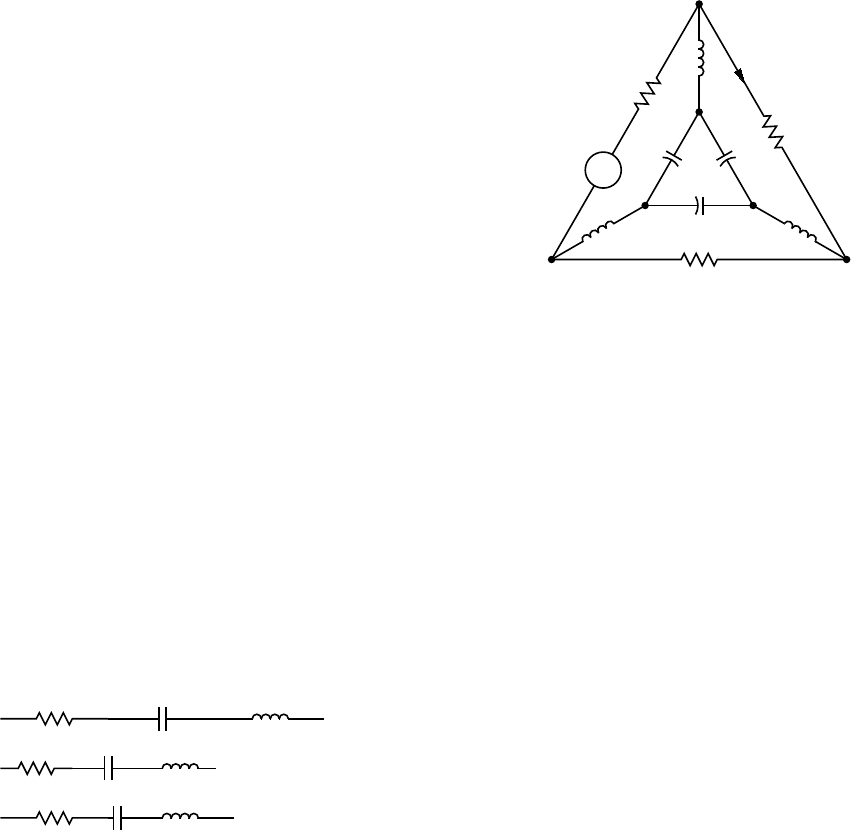.PS
# ‘Oblique.m4’
cct_init
Ct:dot; Point_(-60); capacitor(,C); dlabel(0.12,0.12,,,C_3)
Cr:dot; left_; capacitor(,C); dlabel(0.12,0.12,C_2,,)
Cl:dot; down_; capacitor(from Ct to Cl,C); dlabel(0.12,-0.12,,,C_1)
T:dot(at Ct+(0,elen_))
inductor(from T to Ct); dlabel(0.12,-0.1,,,L_1)
Point_(-30); inductor(from Cr to Cr+vec_(elen_,0))
dlabel(0,-0.1,,L_3,)
R:dot
L:dot( at Cl-(R.x-Cr.x,Cr.y-R.y) )
inductor(from L to Cl); dlabel(0,-0.12,,L_2,)
right_; resistor(from L to R); rlabel(,R_2,)
resistor(from T to R); dlabel(0,0.15,,R_3,) ; b_current(\;y,ljust)
line from L to 0.2<L,T>
source(to 0.5 between L and T); dlabel(sourcerad_+0.07,0.1,-,,+)
resistor(to 0.8 between L and T); dlabel(0,0.15,,R_1,)
line to T
.PE
C
3
C
2
C
1
L
1
L
3
L
2
R
2
R
3
y
+
u
R
1
Figure 20: Illustrating elements drawn at oblique angles.
5.1 Series and parallel circuits
To draw elements in series, each element can be placed by specifying its line segment as described
previously, but the pic language makes some geometries particularly simple. Thus,
setdir_(Right)
resistor; llabel(,R); capacitor; llabel(,C); inductor; llabel(,L)
draws three elements in series as shown in the top line of Figure 21. However, the default length
R
C
L
R
C
L
R
C
L
Figure 21: Three ways of drawing basic elements in series.
elen_
appears too long for some diagrams. It can be redeﬁned temporarily (to
dimen_
, say), by
enclosing the above line in the pair
pushdef(‘elen_’,dimen_) resistor. . . popdef(‘elen_’)
with the result shown in the middle row of the ﬁgure.
Alternatively, the length of each element can be tuned individually; for example, the capacitor in
the above example can be shortened as shown, producing the bottom line of Figure 21:
resistor; llabel(,R)
capacitor(right_ dimen_/4); llabel(,C)
inductor; llabel(,L)
If a macro that takes care of common cases automatically is to be preferred, you can use the
macro
series_(
elementspec, elementspec,